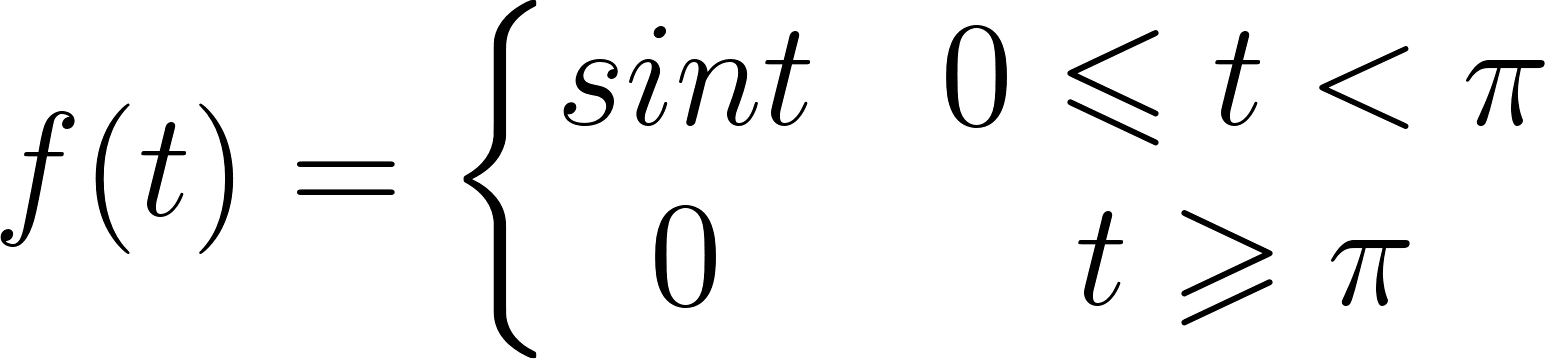# Solved: In 1–18 use Definition 7.1.1 to find {f (t)}.

Chapter 7, Problem 5E

(choose chapter or problem)

Get Unlimited Answers! Check out our subscriptions
QUESTION:

In Problems 1–18 use Definition 7.1.1 to find $$\mathscr{L}\{f(t)\}$$.

$$f(t)=\left\{\begin{array}{lr}\sin t, & 0 \leq t< \\ 0, & t \geq\end{array}\right.$$

Text Transcription:

Lf(t)

f(t)=}lrsin t, & 0 \leq t< \0,&tgeq

QUESTION:

In Problems 1–18 use Definition 7.1.1 to find $$\mathscr{L}\{f(t)\}$$.

$$f(t)=\left\{\begin{array}{lr}\sin t, & 0 \leq t< \\ 0, & t \geq\end{array}\right.$$

Text Transcription:

Lf(t)

f(t)=}lrsin t, & 0 \leq t< \0,&tgeq

Step 1 of 6

In this problem we have to find the Laplace transform of the given function.

Given function isThan definition of Laplace transform gives